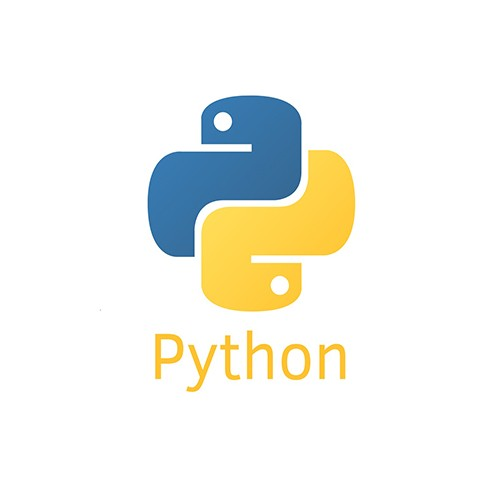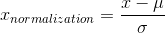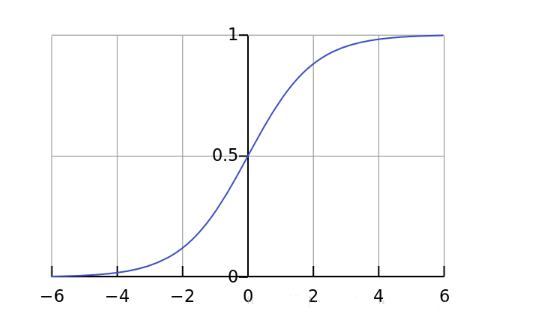# python中怎么进行归一化？• yang   2020-05-22 10:18:37300python实现归一化的方法：

1、(0,1)标准化：

```def MaxMinNormalization(x,Max,Min):
x = (x - Min) / (Max - Min);
return x```

2、Z-score标准化：```def Z_ScoreNormalization(x,mu,sigma):
x = (x - mu) / sigma;
return x```

3、Sigmoid函数：

Sigmoid函数是一个具有S形曲线的函数，是良好的阈值函数，在(0, 0.5)处中心对称，在(0, 0.5)附近有比较大的斜率，而当数据趋向于正无穷和负无穷的时候，映射出来的值就会无限趋向于1和0，是个人非常喜欢的“归一化方法”。```def sigmoid(X,useStatus):
if useStatus:
return 1.0 / (1 + np.exp(-float(X)));
else:
return float(X)```